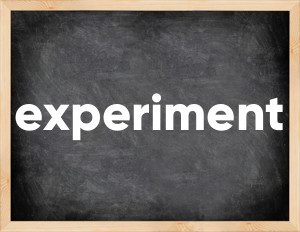# Experiment past tenseThe English verb 'experiment' is pronounced as [ɪkˈsperɪmənt].
Related to: regular verbs.
3 forms of verb experiment: Infinitive (experiment), Past Simple - (experimented), Past Participle - (experimented).

## Here are the past tense forms of the verb experiment

👉 Forms of verb experiment in future and past simple and past participle.
❓ What is the past tense of experiment.

## Experiment: Past, Present, and Participle Forms

Base Form Past Simple Past Participle
experiment [ɪkˈsperɪmənt]

experimented [ɪkˈsperɪməntɪd]

experimented [ɪkˈsperɪməntɪd]

## What are the 2nd and 3rd forms of the verb experiment?

🎓 What are the past simple, future simple, present perfect, past perfect, and future perfect forms of the base form (infinitive) 'experiment'?

### Learn the three forms of the English verb 'experiment'

• the first form (V1) is 'experiment' used in present simple and future simple tenses.
• the second form (V2) is 'experimented' used in past simple tense.
• the third form (V3) is 'experimented' used in present perfect and past perfect tenses.

## What are the past tense and past participle of experiment?

The past tense and past participle of experiment are: experiment in past simple is experimented, and past participle is experimented.

### What is the past tense of experiment?

The past tense of the verb "experiment" is "experimented", and the past participle is "experimented".

### Verb Tenses

Past simple — experiment in past simple experimented (V2).
Future simple — experiment in future simple is experiment (will + V1).
Present Perfect — experiment in present perfect tense is experimented (have/has + V3).
Past Perfect — experiment in past perfect tense is experimented (had + V3).

### experiment regular or irregular verb?

👉 Is 'experiment' a regular or irregular verb? The verb 'experiment' is regular verb.

## Examples of Verb experiment in Sentences

•   We experimented with gas and light weapon (Past Simple)
•   I haven't experimented with this for a long time. (Present Perfect)
•   And besides, he experimented with illegal hallucinogens. (Past Simple)
•   We will experiment with gas weapons. (Future Simple)
•   I read every spellbook I found in my possession, experimented with every potion in my crypt. (Past Simple)
•   She experimented with a pill of some kind that would cut quickly and had a very bad reaction. (Past Simple)
•   I can guess why he is experimenting on my son. (Present Continuous)
•   I experimented during the process and still not happy. (Past Simple)
•   It all started after we experimented with it. (Past Simple)
•   Scientists will start experimenting with electromagnetic pulses. (Future Simple)

Along with experiment, words are popular con into and term.

Verbs by letter: , , , , , , , , , , , , , , , , , , , , , , , , .